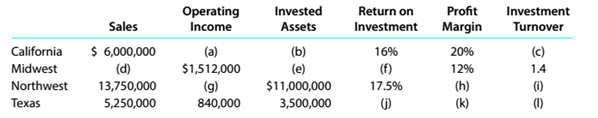Chapter 14, Problem 14.16E

Chapter
Section
Textbook Problem

Determining missing items from computations Data for the California. Midwest, Northwest, and Texas divisions of Firefly Industries are as followsa.Determine the missing items, identifying each by the letters (a) through (I). Round profit margin to one decimal place and investment turnover to two decimal places. b.Determine the residual income for each division, assuming that the minimum acceptable rate of return established by management is 10%. c.Which division is the most profitable in terms of (1) return on investment and (2) residual income?

To determine

(a)

Concept Introduction:

Rate of return on investment represents the investment performance. It is beneficial for investor to compare different investments and select best investment. The benefit or return from the original cost of investment is return on investment.

The missing items in return on investment and residual income computations.

Explanation

California:

Rate of return on investment = Income from operationInvested assets16%=Sales×Profit MarginInvested assets16%=$6,000,000×20%Invested assetsInvested Assets=$1,200,00016%Invested Assets=$7,500,000 Thus, operating income is$1,200,000.

Invested turnover is computed as:

Invested Turnover=SalesInvested assets=$6,000,000$7,500,000=8

Midwest:

Income from operations=Sales×Profit marginSales=Income from operationsProfit margin=$1,512,00012%=12,600,000 Invested asset is computed as: Invested Turnover=SalesInvested assetsInvested Assets=SalesInvested turnover=12,600,0001.4=$9,000,000

Return on investment is as:

Rate of return on investment = Income from operationInvested assets=$1,512,000$9,000,000=16

To determine

(b)

Concept Introduction:

Rate of return on investment represents the investment performance. It is beneficial for investor to compare different investments and select best investment. The benefit or return from the original cost of investment is return on investment.

The residual income assuming the minimum acceptable rate of return is 10%.

To determine

(c)

Concept Introduction:

Rate of return on investment represents the investment performance. It is beneficial for investor to compare different investments and select best investment. The benefit or return from the original cost of investment is return on investment.

The profitable division in term of return on investment and residual income.

Still sussing out bartleby?

Check out a sample textbook solution.

See a sample solution

The Solution to Your Study Problems

Bartleby provides explanations to thousands of textbook problems written by our experts, many with advanced degrees!

Get Started

Find more solutions based on key concepts